# Huffman Coding

Compressing data with binary trees and collections.

Huffman coding is an algorithm devised by David Huffman in 1952 for compressing data, reducing the file size of an image (or any file) without affecting its quality. Unbelievably, this algorithm is still used today in a variety of very important areas. For example, MP3 files and JPEG images both use Huffman coding. We’ll use Huffman coding to compress text files composed of English characters.

## Character representation

Huffman coding relies on the key observation that some characters appear more often than others. The characters that appear most often are, in terms of frequency, more important than the characters that appear less often.

Earlier, we saw that every `char` has an equivalent `int` value between 0 and 255. Computers work with integers in binary format (1’s and 0’s). Binary is similar to normal decimal numbers except it is base2 instead of base10.

For example, the decimal number 120410 is read as 1 · 103 + 2 · 102 + 0 · 101 + 4 · 100. Similarly, we can represent the decimal number 9710 in binary as 011000012 since 9710 is 0 · 27 + 1 · 26 + 1 · 25 + 0 · 24 + 0 · 23 + 0 · 22 + 0 · 21 + 1 · 20.

All Java `char` values can be written using exactly 8 binary digits (bits). This encoding is called ASCII.

The following string data contains 240 characters: 229 a’s, 4 b’s, 3 c’s, and 2 d’s. If each character is represented in Java as an ASCII `char`, then the total file size is 2560 bits.

``````bbbbaaaaaaaaaaaaaaaaaaaaaaaaaaaaaaaaaaaaaaaaaaaaaaaaaaaaaaaaaaaaaaaaaaaaaaaaaaaa
cccaaaaaaaaaaaaaaaaaaaaaaaaaaaaaaaaaaaaaaaaaaaaaaaaaaaaaaaaaaaaaaaaaaaaaaaaaaaaa
ddaaaaaaaaaaaaaaaaaaaaaaaaaaaaaaaaaaaaaaaaaaaaaaaaaaaaaaaaaaaaaaaaaaaaaaaaaaaaaa
``````

However, since a is by far the most frequent character, then it’s better to represent a with the fewest possible bits to save space (followed by b, c, and then d). The following Huffman coding is a more efficient representation of the same string data.

• a can be represented with a one-bit code, 12.
• b can be represented with a two-bit code, 002.
• c can be represented with a three-bit code, 0112.
• d can be represented with a four-bit code, 0102.

The resulting file size is only 255 bits, compressing the data by a factor of 10!

Why not want represent b with a one-bit code, 02?

That could result in ambiguity when decoding the string. For example, given the encoded data, 0112, there are now two possible interpretations!

1. c
2. baa

## Algorithm

Consider the following example found in the `simple-spec-example.txt` file.

``````aba ab cabbb
``````

### Counting characters

The example contains the characters a, b, c, and the space character.

Character’ ‘‘a’‘b’‘c’
Count2451

### Initializing a priority queue

A priority queue will be used to keep track of character counts. Priority queues are a special type of queue ordered by the priority value (e.g. character count) instead of FIFO order, so the `remove` method removes the highest priority element from the queue rather than the oldest element.

In order to represent the (`char`, `int`) pair representing the character together with its frequency, introduce a new data type called `HuffmanNode`. For Huffman coding, rather than remove the highest-frequency character, remove the character with the lowest frequency first.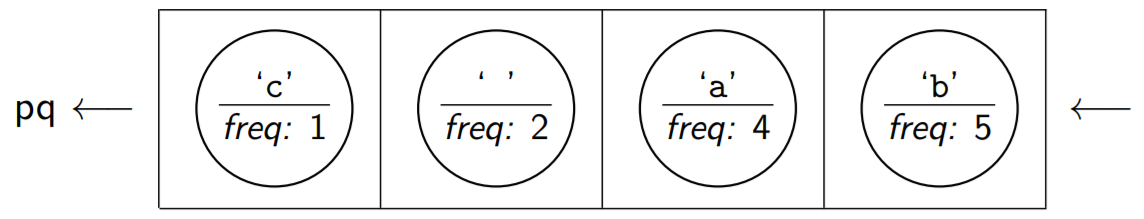Note that the only characters from the file with non-zero frequencies were these characters, so those are the only ones we created HuffmanNodes for

### Combining nodes

Given the priority queue of the nodes, our goal is to form a Huffman tree representing the relative importance of each character. Repeatedly remove the smallest two nodes and create a new node with them as children.

1. First, remove the smallest two nodes from the priority queue.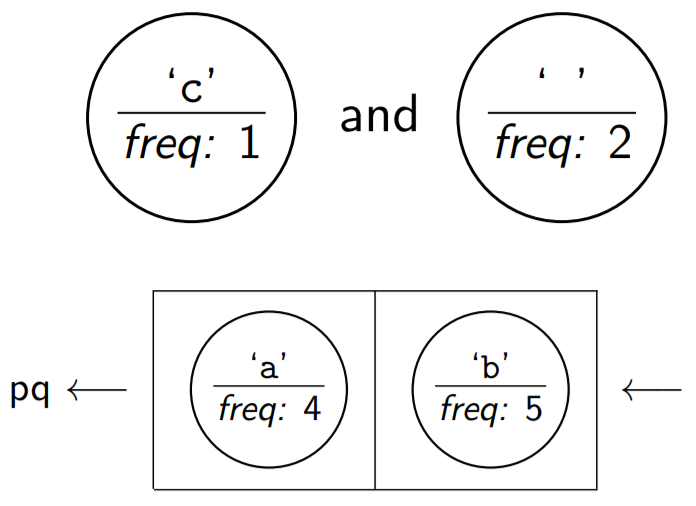2. Then, create a new node. The frequency is the sum of the two nodes.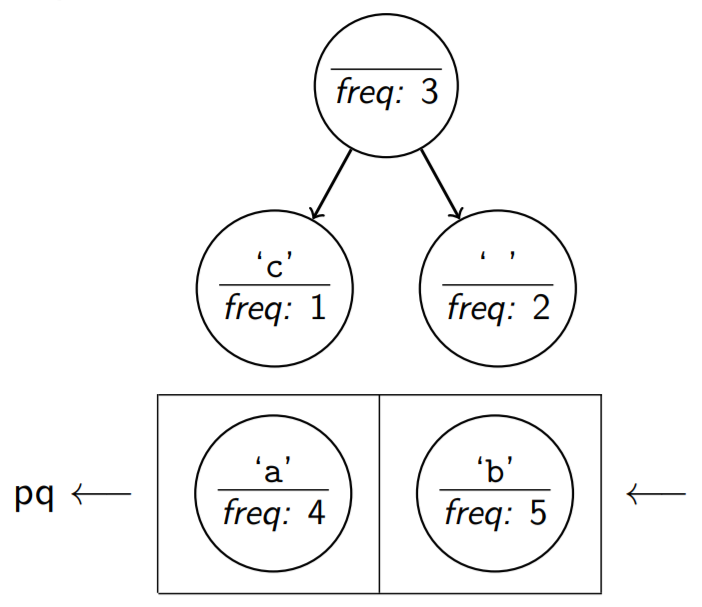3. Add the new node back to the priority queue.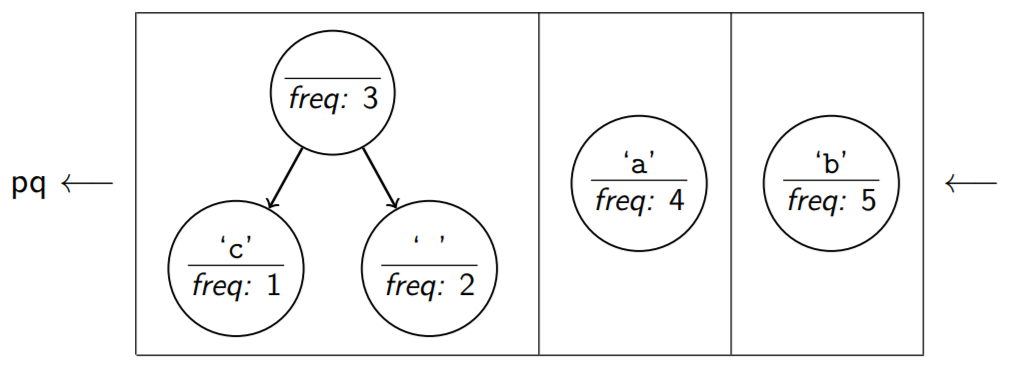Repeat this process until there is only one node in the priority queue representing the complete Huffman tree.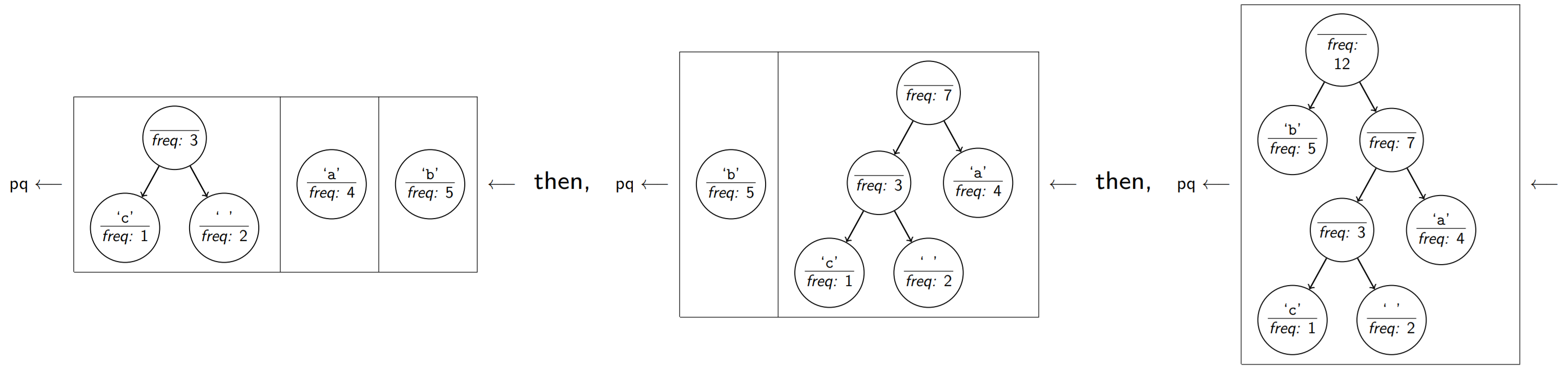### Generating Huffman codes

At this point, the frequencies of the letters have already been taken into account, so character counts are no longer necessary. The resulting Huffman tree represents the optimal encodings for the data file. Notice that the only actual information is in the leaves of the tree.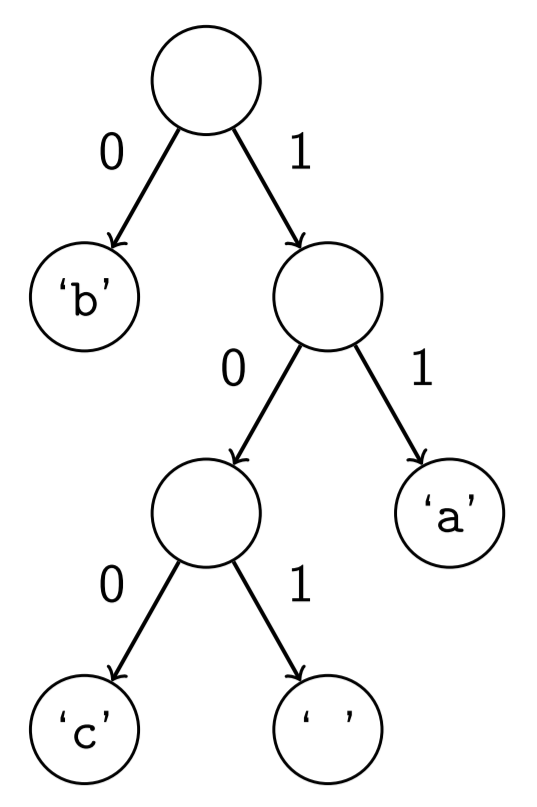To determine the Huffman code for a character, traverse the tree from the root to the node with the letter in it. For left branches, print 0. For right branches, print 1. For example, the character c would be represented with the three-bit code 100, because it is located in the node right, left, left of the overall root.

Output the tree in the following standard `code` file format consisting of pairs of lines, where the first line in the pair is the ASCII value of the character and the second line is the Huffman code for that character. Each pair should appear in traversal order. For example, the `simple-spec-example.code` file contains the following contents.

``````98
0
99
100
32
101
97
11
``````

## HuffmanCode

Implement the `HuffmanCode` class representing a Huffman code for a particular message as a Huffman tree generated according to the described algorithm. The `HuffmanCode` class includes a private static inner class called `HuffmanNode`, representing individual nodes in the Huffman tree.

There are two separate parts to the `HuffmanCode` class.

1. Creating a Huffman code with the `frequencies` constructor and `save` method.
2. Decompressing a message with the `input` constructor and `translate` method.

### HuffmanNode

The contents of the `HuffmanNode` class are up to you, but it should have at least one constructor used by the `HuffmanCode` class and all node fields must be declared public. `HuffmanNode` should not contain any of the actual algorithm.

Since the priority queue will ultimately be declared as `PriorityQueue<HuffmanNode>`, the `HuffmanNode` class must implement `Comparable<HuffmanNode>`. Use the frequency of the subtree to determine its ordering relative to other subtrees, with lower frequencies considered “less than” higher frequencies. If two frequencies are equal, the nodes are considered equal as well.

### Method summary

`public HuffmanCode(int[] frequencies)`
Initializes a new `HuffmanCode` object using the described algorithm given an array of `frequencies` where `frequences[i]` represents the count of the character with ASCII value `i`. Use a `PriorityQueue` to build the Huffman code.
`public HuffmanCode(Scanner input)`
Initializes a new `HuffmanCode` object by reading in a previously saved `code` file. Assume the `Scanner` is not `null` and always contains data encoded in the standard format. Since frequencies are irrelevant for this part, all node frequencies should be set to some standard value such as 0 or -1.
`public void save(PrintStream output)`
Stores the current Huffman codes to the given `output` stream in the standard format.
`public void translate(BitInputStream input, PrintStream output)`
Reads individual bits from the `input` stream and writes the corresponding characters to the `output`. Stops reading when the `input` is empty. Assume the `input` stream is compatible with this Huffman tree.

### Line-based processing

When implementing the `Scanner` constructor, do not call `nextInt()` to read the integer and `nextLine()`. Mixing token-based reading and line-based reading can cause problems. Instead, use only line-based reading and call `Integer.parseInt` when `int` values are needed.

``````int asciiValue = Integer.parseInt(input.nextLine());
String code = input.nextLine();
``````

### Translate example

Algorithm for `translate`
1. Begin at the top of the tree.
2. For each bit in the `input`, go left for 0 and go right for 1.
3. At a leaf node, `write` the integer code to the `output`.
4. Repeat this process so long as there are still more bits to read.

Suppose we have the following `simple-spec-example.short` message encoded using the `simple-spec-example.code` Huffman coding.

``````1101110111010110011000
``````

The trace below diagrams the steps determining each character in the `short` message, resulting in the decompressed message, “aba ab cabbb”.

1. Read 1, go right. Read 1, go right. ‘a’ is a leaf. Output ‘a’. Remaining input is 01110111010110011000.
2. Read 0, go left. ‘b’ is a leaf. Output ‘b’. Remaining input is 1110111010110011000.
3. Read 1, go right. Read 1, go right. ‘a’ is a leaf. Output ‘a’. Remaining input is 10111010110011000.
4. Read 1, go right. Read 0, go left. Read 1, go right. ‘ ‘ is a leaf. Output ‘ ‘. Remaining input is 11010110011000.
5. Read 1, go right. Read 1, go right. ‘a’ is a leaf. Output ‘a’. Remaining input is 010110011000.
6. Read 0, go left. ‘b’ is a leaf. Output ‘b’. Remaining input is 10110011000.
7. Read 1, go right. Read 0, go left. Read 1, go right. ‘ ‘ is a leaf. Output ‘ ‘. Remaining input is 10011000.
8. Read 1, go right. Read 0, go left. Read 0, go right. ‘c’ is a leaf. Output ‘c’. Remaining input is 11000.
9. Read 1, go right. Read 1, go right. ‘a’ is a leaf. Output ‘a’. Remaining input is 000.
10. Read 0, go left. ‘b’ is a leaf. Output ‘b’. Remaining input is 00.
11. Read 0, go left. ‘b’ is a leaf. Output ‘b’. Remaining input is 0.
12. Read 0, go left. ‘b’ is a leaf. Output ‘b’. Remaining input is empty.

When implementing `translate`, do not call `System.out.print` and instead `write` to the given `output`. The `PrintStream` class contains a `write` method that accepts an `int` as a parameter. Be careful with using recursion since Java may throw a `StackOverflowError` when it exceeds a certain number of recursive calls. For a large message, there may be hundreds of thousands of characters to decode.

## BitInputStream

The provided `BitInputStream` class reads data bit-by-bit for the `translate` method in `HuffmanCode`. The interface for `BitInputStream` looks very much like a `Scanner`, so it should be used in a similiar fashion.

`public int nextBit()`
Returns the next bit in the input stream. Throws a `NoSuchElementException` if there are no more bits.
`public boolean hasNextBit()`
This method returns true if the input stream has at least one more bit and false otherwise.

Avoid unnecessary logic such as extra base cases or recursive cases. Use the `x = change(x)` pattern to simplify code and use the Output Comparison Tool to check the output format! Remember to declare variables with their interface types when possible, so `PriorityQueue` variables should be delcared using the `Queue` interface type.

The Huffman coding for a given message is not necessarily unique. If implemented exactly as specified, then you should get exactly the same tree so long as, when combining nodes, the first node removed becomes the left subtree and the second node removed becomes the right subtree.

## Reflection

Create a new file `reflection.txt` and write a paragraph-long reflection answering the following questions (along with anything else you find appropriate):

1. What did you learn this week?
2. What did you enjoy in the course this week?
3. What did you find challenging or frustrating this week?
4. What did you find particularly helpful for your learning this week?

## Submission

In addition to the completed program, submit files named `secretmessage.short` and `secretmessage.code` that represent a secret compressed message from you to your TA. The message can be anything you want as long as it is not offensive.

Submit `HuffmanCode.java`, `secretmessage.short`, `secretmessage.code`, and `reflection.txt` to Grade-It!